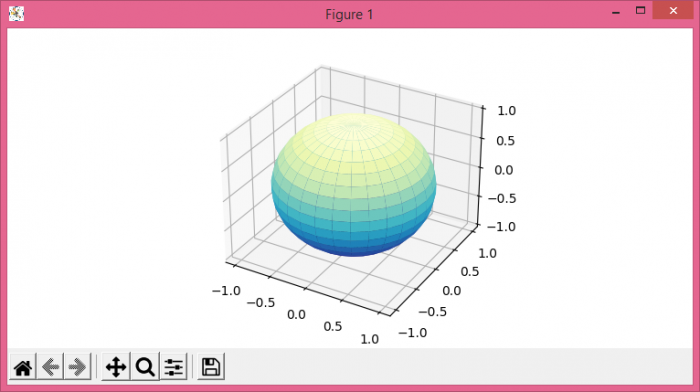# What is the equivalent of Matlab's surf(x,y,z,c) in Matplotlib?

Let's take an example to see how to get the same effect as MatLab's surf(x,y,z,c) in Matplotlib. steps −

• Set the figure size and adjust the padding between and around the subplots.

• Create a new figure or activate an existing figure.

• Add an 'ax' to the figure as part of a subplot arrangement.

• Create r, u, v, x, y and z data points using Numpy.

• Create a surface plot.

• To display the figure, use show() method.

## Example

import matplotlib.pyplot as plt
import numpy as np

plt.rcParams["figure.figsize"] = [7.50, 3.50]
plt.rcParams["figure.autolayout"] = True

fig = plt.figure()
r = 0.05
u, v = np.mgrid[0:2 * np.pi:30j, 0:np.pi:20j]

x = np.cos(u) * np.sin(v)
y = np.sin(u) * np.sin(v)
z = np.cos(v)

ax.plot_surface(x, y, z, cmap=plt.cm.YlGnBu_r)

plt.show()

## Output